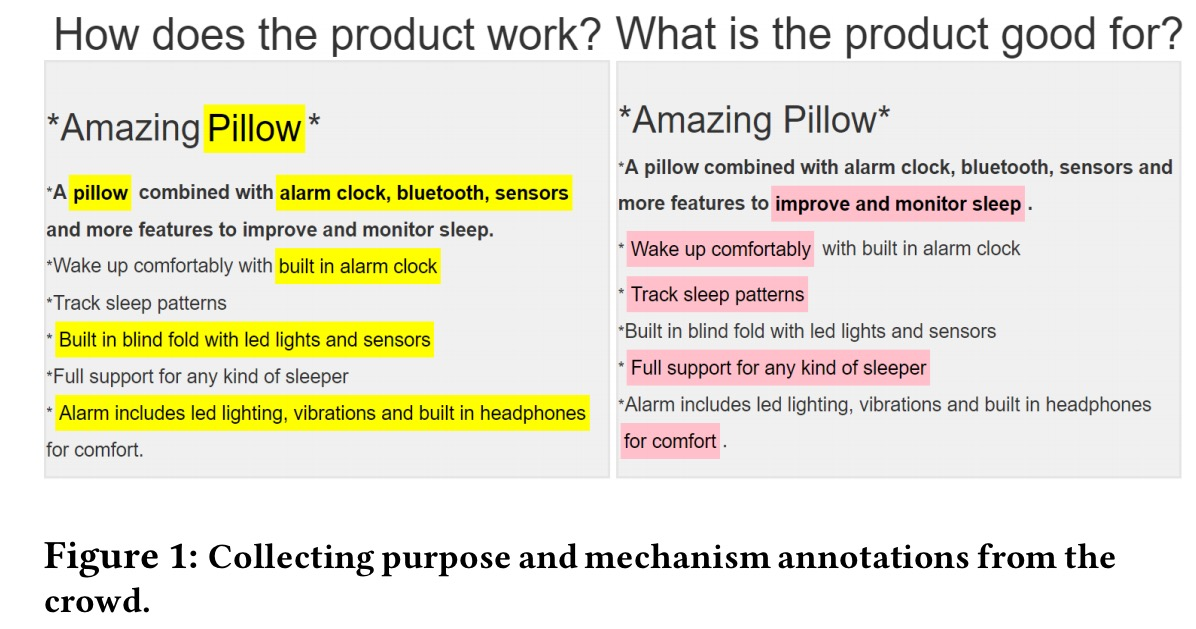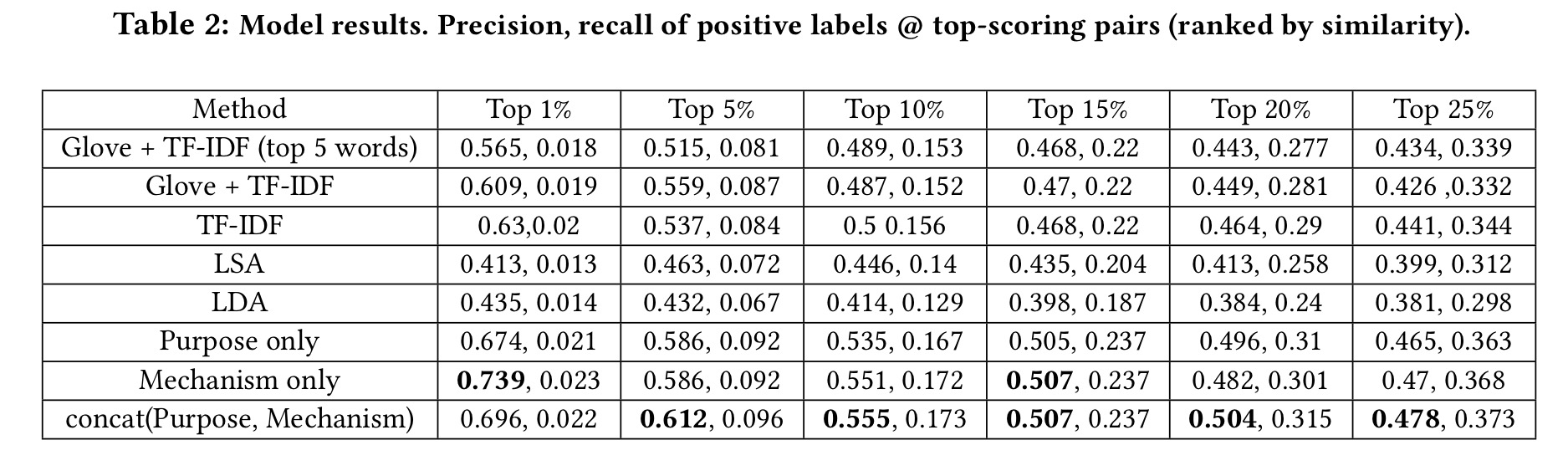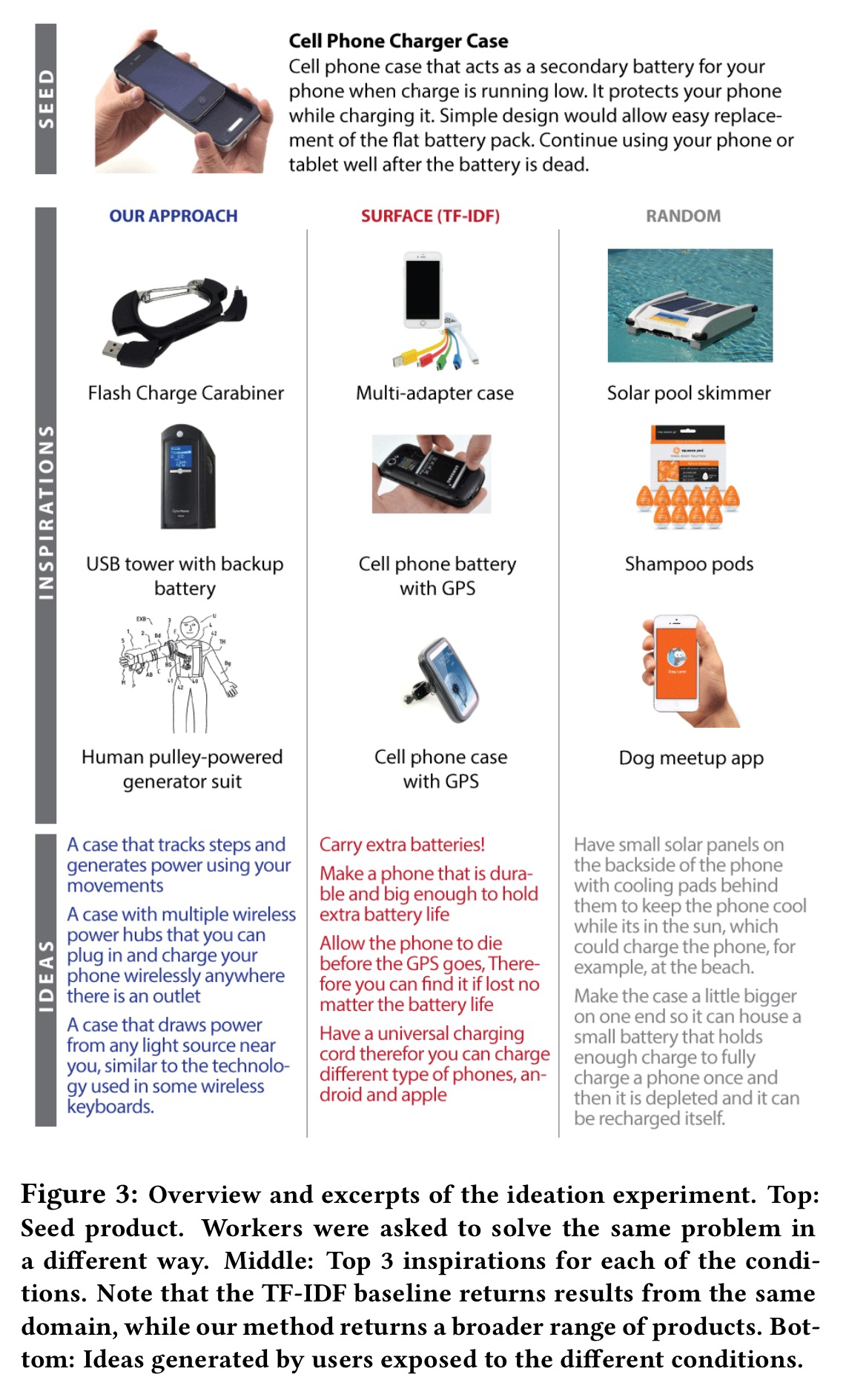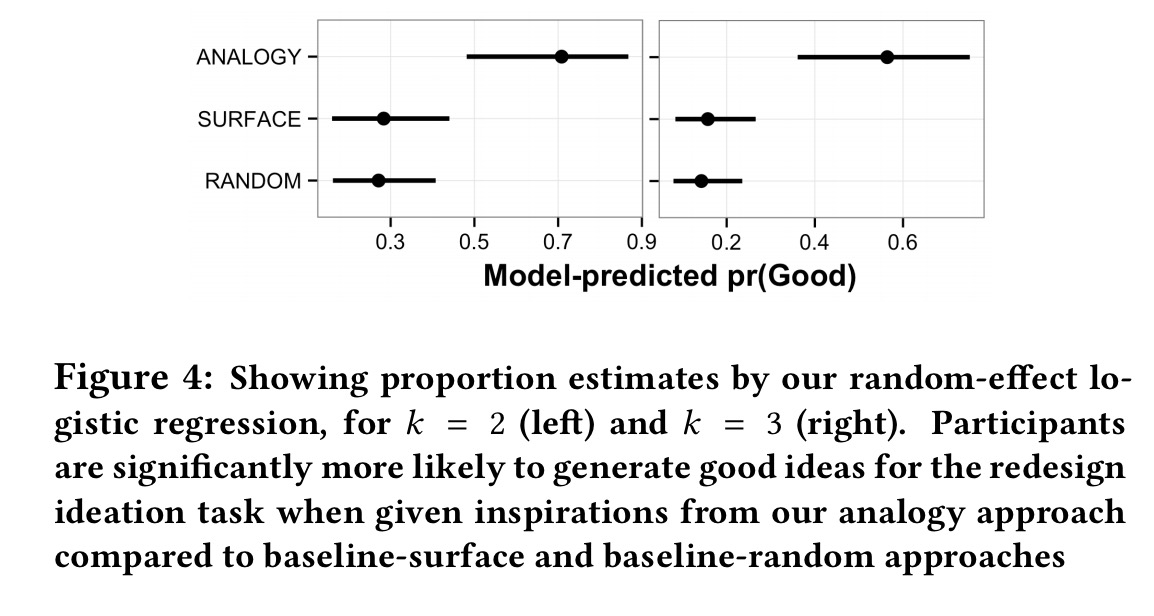# Accelerating innovation through analogy mining

Accelerating innovation through analogy mining Hope et al., KDD’17

Today’s choice won a best paper award at KDD’17. It’s a really interesting twist on information retrieval, building on a foundation of GloVe and word vectors to create purpose and mechanism vectors for a corpus of product descriptions. Using these vectors, the authors show how to search for products with similar purpose but different mechanisms. The search results are used in ideation experiments to help people generate creative ideas, and proved better for this purpose than the alternatives. It’s the vector-based information retrieval parts that I find the most interesting though, in a similar manner to ‘Using word embedding to enable semantic queries on relational databases.’

We imagine a future in which people could search through data based on deep analogical similarity rather than simple keywords; lawyers or legal scholars could find legal precedents sharing similar systems of relations to a contemporary case; and product or service designers could mine myriad potential solutions to their problem.

Finding analogies is challenging, since most existing approaches detect surface similarities rather than any understanding of deep relational similarity.

### Purpose and mechanism

If you want to find good analogous examples, where do you start? Analogies are linked to purpose – what a product does or is used for – and to mechanism – how it does it.

The importance of a product’s purpose and mechanism as core components of analogy are theoretically rooted in early cognitive psychology work on schema induction which define the core components of a schema as a goal and proposed solution to it.

Suppose for each product$i \in \mathcal{P}$ we had purpose and mechanism vectors$\mathbf{p}_i$,$\mathbf{m}_i$, and we also have distance metrics$d_p(\dot,\dot)$,$d_m(\dot,\dot)$ between purpose and mechanism vectors respectively.

Then we could for example search for products that have the same (very similar) purpose, but a different mechanism. We want to find a product with the most similar purpose,$\underset{\tilde{i} \in \mathcal{P}}{\mathrm{argmin}}\ d_p(\mathbf{p}_i, \mathbf{p}_{\tilde{i}})$, subject to the mechanism being sufficiently different:$d_m(\mathbf{m}_i, \mathbf{m}_{\tilde{i}}) \geq threshold$.

We could also search for products using the same (very similar) mechanism, but for a different purpose. We want to find a product with a similar mechanism,$\underset{\tilde{i} \in \mathcal{P}}{\mathrm{argmin}}\ d_m(\mathbf{m}_i, \mathbf{m}_{\tilde{i}})$ subject to the purpose being sufficiently different:$d_p(\mathbf{p}_i, \mathbf{p}_{\tilde{i}}) \geq threshold$.

That looks like it would be pretty nifty. If only there was a way to obtain such product and mechanism vectors…

### Extracting purpose and mechanism vectors

The base corpus used in the study is a collection of 8,500 product ideas from Quirky.com. The ideas are unstructured and cover a variety of domains, making cross-domain analogies possible.

Here’s an example for a “my pet is thirsty too” in-car pet bowl:Amazon Mechanical Turk crowd workers were used to annotate purpose words and phrases and mechanism words and phrases in each of the product descriptions. Each product was annotated by four workers, using a UI that placed purpose and mechanism side-by-side to discourage overlapping tags.If it all seems a little bit haphazard and imprecise, that’s kind of the point – even from such data it turns out we can do useful things.

From this markup, we start by extracting binary vectors for product and mechanism. The purpose binary vector has one entry for every word in the product description, with value 1 if it was annotated as a purpose word, and 0 otherwise. The mechanism vector is constructed in a similar manner. There will be K (4) of these for each product, one for each worker. These binary vectors are somewhat analogous to one-hot word vectors at this point – no use for comparing between vectors beyond equality. Let’s add some meaning

For each word in the product description, take the GloVe word vector for that word (from a set of vectors pre-trained on the Common Crawl web data). Now form a sequence of word vectors for the words with bit set to 1 in the purpose binary vector. Compute the TF-IDF scores for the tokens in the sequence and find the D (= 5) tokens with the top TF-IDF scores. These will be the D most unique purpose words. The final purpose vector is formed by taking TF-IDF weighted average of the corresponding D GloVe vectors. We can do similarly for mechanism. In the study, the authors use vectors of dimension 300.

### Predicting purpose and mechanism for new examples

Repeat the above process for each of the N training examples, and you end up with a set of N target tuples$\{(\mathbf{p}_1,\mathbf{m}_1), (\mathbf{p}_2,\mathbf{m}_2), ..., (\mathbf{p}_N,\mathbf{m}_N)\}$. We want to learn a function that predicts$(\mathbf{p}_i, \mathbf{m}_i)$ given as input a variable length sequence$\mathbf{w}_i$ comprising the GloVe word vectors for each word in the product description.

This function is learned using a bi-direction RNN passing forward and backward over the word vector sequence$\mathbf{w}_i$. If$\mathbf{W}_p$ and$\mathbf{W}_m$ are purpose and weight vectors, and$h$ is the hidden representation, then:$\hat{\mathbf{p}_i} = \mathbf{W}_{p}h_i^T, \hat{\mathbf{m}_i} = \mathbf{W}_{m} h_i^T$.

### Generating analogies: foundation

Amazon Mechanical Turk workers were used to create a seed set of 200 product pairs of analogous products. That’s not a perfect process, but the authors designed a custom ‘analogy search’ interface to help the workers find matches. Examples tagged as matches became positive examples in the analogy dataset, examples rejected during the search became negative examples.

All pairs of products in the test data were then ranked according to a number of criteria, including use of the purpose and mechanism vectors, to see which methods gave the best precision and recall for analogous pairs. The results show that the purpose and mechanism vectors do indeed seem to be well suited for this task.### Generating near-purpose, far-mechanism analogies

So now we come to the challenge of using the vectors to help with ideation – we want to find pairs of products with near purpose, but far apart mechanisms.

The first step is to run a k-means clustering algorithm over the purpose vectors, and then prune away the smaller clusters. The remaining clusters are ranked by an intra-distance measure and the top$k$ are selected. For each of these top purpose clusters, select the product with purpose vector closest to the centre of the cluster. Use this product as the kth seed product,$s_k$.

For each of these seed products, the other members from the same cluster are all candidate matches with a similar purpose. Some may have fairly similar mechanism vectors, and some less so. We want to ensure good diversity in the set of results obtained.

The problem of extracting a well-diversified subset of results from a larger set of candidates has seen a lot of work, prominently in the context of information retrieval (which is closely related to our setting)… There are many ways to diversify results, mainly differing by objective function and constraints. Two canonical measures are the MAX-MIN and MAX-AVG dispersion problems.

The authors go with MAX-MIN, which looks to maximise the minimum distance between all pairs in the set.

### Are the analogies useful for generating ideas?

The authors carefully design a study comparing the usefulness of the suggestions in creating good ideas. Suggestions created using the near-purpose, far-mechanism analogy generation process are compared with a baseline of suggestions found using TF-IDF (simulating current search engines), and a purely random selection of products from the corpus. Teams of human judges are used to decide whether or not the ideas generated by the participants were in fact ‘good.’ An idea is good if it (1) uses a different mechanism or technology than the original product, (2) achieves the same purpose as the original product, and (3) can be implemented using existing technology.

Here’s an example of a product shown to study participants, and the suggestions the various mechanisms produced to help them generate new ideas. In many ways, this is my least favourite part of the paper, because the suggestions from the analogy mechanism in this instance don’t seem obviously better than those in the other columns. Or is it just me?Regardless, when the analysis is done over the whole study, participants using the analogy-driven suggestions turn out to be much more likely to generate good ideas. In the figure below k=2 refers to 2 out of 3 judges agreeing an idea is good, and k=3 means that all 3 judges thought an idea was good.The takeaway from this study is that our approach yields a reliable increase in participants’ creative ability.

The main takeaway from the paper for me though, is the use of semantic vectors extracted from unstructured text to aid in information retrieval tasks.